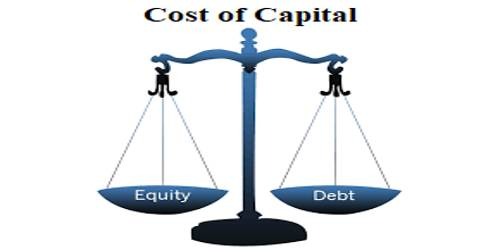# How to estimate the Cost of Equity Capital?

Estimate the Cost of Equity Capital –

The cost of capital or required rate for return a firm can be defined as the composite cost of the firm’s financing components. The cost of capital is the rate of return a firm must earn on its investments in the project in order to maintain the market value of its. It is rather complicated to estimate because there is no exact commitment on the part of the company to pay dividends.

The cost of equity is the return that stockholders require for their investment in a company. The cost of equity is the rate of return required to persuade an investor to make a given equity investment. The traditional formula for the cost of equity (COE) is the dividend capitalization model:

Cost of Equity = [Dividends per Share (for next year) / Current Market Value of Stock]

The cost of equity can also be calculated by using the CAPM (Capital Asset Pricing Model) or Dividend Capitalization Model (for companies that pay out dividends).

A firm’s cost of equity represents the compensation that the market demands in exchange for owning the asset and bearing the risk of ownership. A firm uses the cost of equity to assess the relative attractiveness of investments, including both internal projects and external acquisition opportunities.